• 取n阶矩阵A的特征值，相对应的特征向量线性无关， 即有： 令，则P非奇异 所以 等式两边同时左乘可得     必要性 取n阶矩阵A与对角矩阵相似，则存在非奇异矩阵使 等式两边同时左乘P可得 即 ...
证明：
充分性
取n阶矩阵A的特征值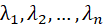，相对应的特征向量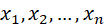线性无关，
即有：

令，则P非奇异

所以 等式两边同时左乘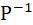可得

必要性
取n阶矩阵A与对角矩阵相似，则存在非奇异矩阵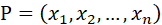使

等式两边同时左乘P可得

即

所以

因此，P的列向量就是其特征值λi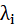对应的特征向量，由于P非奇异，线性无关。


展开全文• 线性代数之线性相关线性表示的求法 线性相关 向量是n个m维(每个向量分量的个数)的向量，若存在一组不全为0的 使得 则 是线性相关的，反之线性无关。... 一个向量线性相关的充要条件是向量为0向量 线性表示...
线性代数之线性相关线性表示的求法
线性相关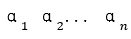向量是n个m维(每个向量分量的个数)的向量，若存在一组不全为0的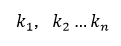使得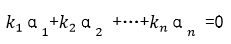则是线性相关的，反之线性无关。
线性无关即等价于以下命题：
线性不相关找不到一组不全0的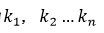使得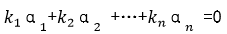全为0
几种情况:
关于单个向量
向量组中两个向量成比例，则两个向量必线性相关含零向量的任向量组必线性相关(取0向量的系数为1或者k，其余均为0)一个零向量必线性相关一个非零向量必然线性无关一个向量线性相关的充要条件是向量为0向量
线性表示
如果向量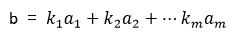则b是向量组A的线性组合，这是向量b可有向量组A线性表示。这里其实转换为了方程有解，全是0也是有解。
特别的：
线性表示时系数可以全是00向量可有任意向量组表示。
任何向量都可由 (1,0,...0),(0,1,0...0),(0,0,1...0) ...(0,0...0...1)表示

线性相关例子汇总
判断线性相关（不含参数）
该方法是根据矩阵的秩的定义来求，如果找到k阶子式为0，而k-1阶不为0，那么k-1即该矩阵的秩。
#Sample1（示例一）,判断如下向量组是否线性相关：
1：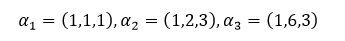2：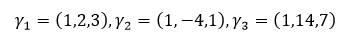解：针对第一题：
Step1：首先我们先立方程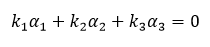针对其解的情况来判断向量组是否线性相关(有解)或者无关（无解）。
Step2：于是我们得到下式：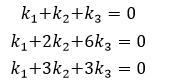Step3： 我们对k的行列式化简得到如下行列式：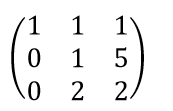该行列式不为0，所以当前关于k的方程组有唯一解，即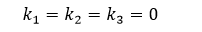所以当前向量组里的向量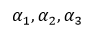线性无关。
针对第二题：同样的思路
Step1：设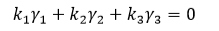Step2：于是我们得到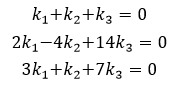Step3：针对k化简得到如下行列式，易得其为0,所以k有非零解。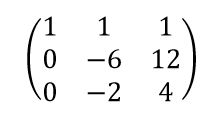Step4：因为关于k的解有无穷个，所有这里取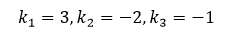换言之存在不全为0的数使得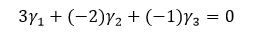即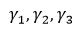线性相关。
判断线性相关(含参数)
针对这种类型的问题，一般将它们按照列(行)的形式构成矩阵，对矩阵做行(列)变换，使矩阵变成阶梯型。最后根据矩阵中参数的取值是否使得其所在行（列）为零行来判断向量组的线性相关性。（参数所在行全为0则行列式为0，线性无关，否则相关）。
#Sample2（示例二）：已知向量组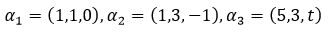判断其相关性。
解：
Step1：因这里向量组的向量个数和向量的维数相同，所以可以按照列组成行列式。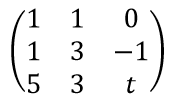Step2：第1行的-1倍加到第2行上去，第1行的-5倍加到第3行上去，则得: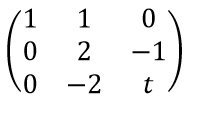即行列式等于2(t-1)
Step3:针对Step2里的t进行讨论，如果t=1，则行列式等于0(即方程有无穷非非零解)，则线性相关，如果t≠1则行列式不等于0（即方程只有零解），则线性无关。
线性表示例子汇总
阶梯法判断线性表示
利用矩阵的初等变换不改变矩阵的列的线性关系的特点求解。
#Sample3（示例三）
向量β=（4,4,1，2）是否可由如下向量组线性表示，如果可以，写出表达式。
1: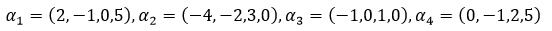2: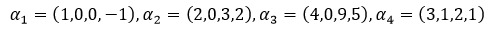解：
针对第一题：
Step1：用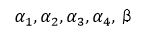作为列向量构成矩阵A，则A为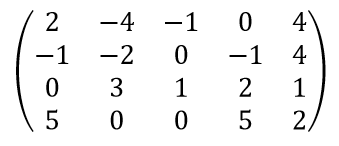Step2：交换第1和第2行，则化为：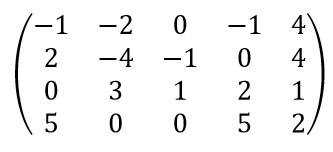Step3：第1行的2倍加到第2行上去，第1行的5倍加到第4行上去，第1行乘-1，则最终化为：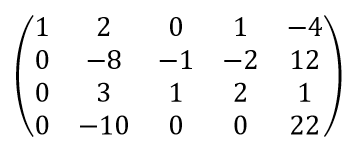Step4：在对step3里的矩阵化简，第3行的3倍加到第2、4行上去，则得：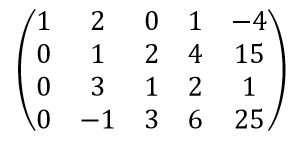Step5：在对step4里的矩阵化简，第2行的-3倍加到第3行上去，第2行的1倍加到第3行上去，则得：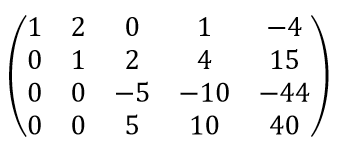Step6：在对step5里的矩阵化简，第3行的1倍加到第4行上去，第3行除以-5，则得：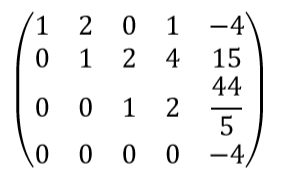Step7：由A的阶梯型可知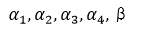这5个向量的向量组的秩（阶梯型里非零行的行数）是4，所以该向量组的秩必定包含β，即β不能由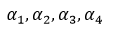线性表示。

针对第二题：
类似第一题，可将构成的矩阵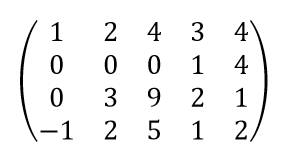化简为：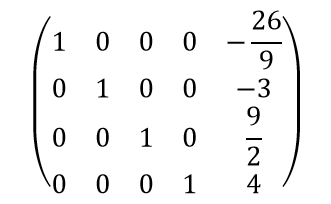则可见即β可由线性表示，即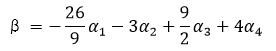展开全文• 特征向量线性无关性 设 λ1,⋯,λm" role="presentation" style="position: relative;">λ1,⋯,λmλ1,⋯,λm\lambda_1, \cdots, \lambda_m 是线性变换 f" role="presentation" style=...
特征向量的线性无关性
设

λ1,⋯,λm

λ

1

,

⋯

,

λ

m

$\lambda_1, \cdots, \lambda_m$ 是线性变换

f

f

$f$ 的 m$m$$m$ 个不同的特征值，

ξi1,⋯,ξini

ξ

i

1

,

⋯

,

ξ

i

n

i

$\xi_{i1}, \cdots, \xi_{in_i}$ 是属于

λi

λ

i

$\lambda_i$ 的

ni

n

i

$n_i$ 个线性无关的特征向量，  则所有这些这些向量组成的向量组：

ξ11,⋯,ξ1n1,⋯,ξm1,⋯,ξmnm

ξ

11

,

⋯

,

ξ

1

n

1

,

⋯

,

ξ

m

1

,

⋯

,

ξ

m

n

m

$\xi_{11}, \cdots, \xi_{1n_1}, \cdots, \xi_{m1}, \cdots, \xi_{mn_m}$也线性无关。
证明
命题就是：

∑i=1m∑j=1nikijξij=0⃗ ⇒kij=0,i,j∈N,1≤i≤m,1≤j≤ni,

∑

i

=

1

m

∑

j

=

1

n

i

k

i

j

ξ

i

j

=

0

→

⇒

k

i

j

=

0

,

i

,

j

∈

N

,

1

≤

i

≤

m

,

1

≤

j

≤

n

i

,

$\sum \limits_{i = 1} ^{m} \sum \limits_{j = 1} ^{n_i}k_{ij} \xi_{ij} = \vec {0} \Rightarrow k_{ij} = 0, i, j \in \mathbb N, 1 \le i \le m, 1 \le j \le n_i,$

m=1

m

=

1

$m = 1$ 时命题显然成立。  假设

m

m

$m$ 时命题成立。则 m+1$m+1$$m + 1$ 时：  设

∑i=1m+1∑j=1nikijξij=0⃗

∑

i

=

1

m

+

1

∑

j

=

1

n

i

k

i

j

ξ

i

j

=

0

→

$\sum \limits_{i = 1} ^{m + 1} \sum \limits_{j = 1} ^{n_i}k_{ij} \xi_{ij} = \vec {0}$  则

f(∑i=1m+1∑j=1nikijξij)=f(0⃗ )=0⃗

f

(

∑

i

=

1

m

+

1

∑

j

=

1

n

i

k

i

j

ξ

i

j

)

=

f

(

0

→

)

=

0

→

$f (\sum \limits_{i = 1} ^{m + 1} \sum \limits_{j = 1} ^{n_i}k_{ij} \xi_{ij} )= f(\vec {0}) = \vec {0}$

⇒∑i=1m+1∑j=1nikijf(ξij)=0⃗

⇒

∑

i

=

1

m

+

1

∑

j

=

1

n

i

k

i

j

f

(

ξ

i

j

)

=

0

→

$\Rightarrow \sum \limits_{i = 1} ^{m + 1} \sum \limits_{j = 1} ^{n_i}k_{ij} f( \xi_{ij} ) = \vec {0}$

⇒∑i=1m+1∑j=1nikijλiξij=0⃗

⇒

∑

i

=

1

m

+

1

∑

j

=

1

n

i

k

i

j

λ

i

ξ

i

j

=

0

→

$\Rightarrow \sum \limits_{i = 1} ^{m + 1} \sum \limits_{j = 1} ^{n_i}k_{ij} \lambda_i \xi_{ij} = \vec {0}$

⇒∑i=1m+1λi∑j=1nikijξij=0⃗

⇒

∑

i

=

1

m

+

1

λ

i

∑

j

=

1

n

i

k

i

j

ξ

i

j

=

0

→

$\Rightarrow \sum \limits_{i = 1} ^{m + 1} \lambda_i \sum \limits_{j = 1} ^{n_i}k_{ij} \xi_{ij} = \vec {0}$  又

λm+1∑i=1m+1∑j=1nikijξij=0⃗

λ

m

+

1

∑

i

=

1

m

+

1

∑

j

=

1

n

i

k

i

j

ξ

i

j

=

0

→

$\lambda_{m + 1} \sum \limits_{i = 1} ^{m + 1} \sum \limits_{j = 1} ^{n_i}k_{ij} \xi_{ij} = \vec {0}$

⇒∑i=1m+1λm+1∑j=1nikijξij=0⃗

⇒

∑

i

=

1

m

+

1

λ

m

+

1

∑

j

=

1

n

i

k

i

j

ξ

i

j

=

0

→

$\Rightarrow \sum \limits_{i = 1} ^{m + 1} \lambda_{m + 1} \sum \limits_{j = 1} ^{n_i}k_{ij} \xi_{ij} = \vec {0}$  因此

∑i=1m+1(λi−λm+1)∑j=1nikijξij=0⃗

∑

i

=

1

m

+

1

(

λ

i

−

λ

m

+

1

)

∑

j

=

1

n

i

k

i

j

ξ

i

j

=

0

→

$\sum \limits_{i = 1} ^{m + 1} (\lambda_i - \lambda_{m + 1}) \sum \limits_{j = 1} ^{n_i}k_{ij} \xi_{ij} = \vec {0}$

⇒∑i=1m(λi−λm+1)∑j=1nikijξij=0⃗

⇒

∑

i

=

1

m

(

λ

i

−

λ

m

+

1

)

∑

j

=

1

n

i

k

i

j

ξ

i

j

=

0

→

$\Rightarrow \sum \limits_{i = 1} ^{m} (\lambda_i - \lambda_{m + 1}) \sum \limits_{j = 1} ^{n_i}k_{ij} \xi_{ij} = \vec {0}$

⇒∑i=1m∑j=1ni(λi−λm+1)kijξij=0⃗

⇒

∑

i

=

1

m

∑

j

=

1

n

i

(

λ

i

−

λ

m

+

1

)

k

i

j

ξ

i

j

=

0

→

$\Rightarrow \sum \limits_{i = 1} ^{m}\sum \limits_{j = 1} ^{n_i} (\lambda_i - \lambda_{m + 1}) k_{ij} \xi_{ij} = \vec {0}$  由归纳假设，

(λi−λm+1)kij=0,i,j∈N,1≤i≤m,1≤j≤ni,

(

λ

i

−

λ

m

+

1

)

k

i

j

=

0

,

i

,

j

∈

N

,

1

≤

i

≤

m

,

1

≤

j

≤

n

i

,

$(\lambda_i - \lambda_{m + 1}) k_{ij} = 0, i, j \in \mathbb N, 1 \le i \le m, 1 \le j \le n_i,$  由于

λi≠λm+1,i,∈N,1≤i≤m,

λ

i

≠

λ

m

+

1

,

i

,

∈

N

,

1

≤

i

≤

m

,

$\lambda_i \neq \lambda_{m + 1}, i, \in \mathbb N, 1 \le i \le m,$ 因此

kij=0,i,j∈N,1≤i≤m,1≤j≤ni,

k

i

j

=

0

,

i

,

j

∈

N

,

1

≤

i

≤

m

,

1

≤

j

≤

n

i

,

$k_{ij} = 0, i, j \in \mathbb N, 1 \le i \le m, 1 \le j \le n_i,$  于是

∑j=1nm+1k(m+1)jξ(m+1)j=0⃗

∑

j

=

1

n

m

+

1

k

(

m

+

1

)

j

ξ

(

m

+

1

)

j

=

0

→

$\sum \limits_{j = 1} ^{n_{m + 1}}k_{{(m + 1)}j} \xi_{{(m + 1)}j} = \vec {0}$

⇒k(m+1)j=0,j∈N,1≤j≤nm+1,

⇒

k

(

m

+

1

)

j

=

0

,

j

∈

N

,

1

≤

j

≤

n

m

+

1

,

$\Rightarrow k_{{(m + 1)}j} = 0, j \in \mathbb N, 1 \le j \le n_{m + 1},$  因此

kij=0,i,j∈N,1≤i≤m,1≤j≤ni,

k

i

j

=

0

,

i

,

j

∈

N

,

1

≤

i

≤

m

,

1

≤

j

≤

n

i

,

$k_{ij} = 0, i, j \in \mathbb N, 1 \le i \le m, 1 \le j \le n_i,$
展开全文• n维列向量组α1,α2,...,αm,m, \alpha_2,...,\alpha_m, m 线性无关，则n维列向量组β1,β2,...,βm\beta_1,\beta_2,...,\beta_m线性无关充要条件是（D） A. 向量组α1,α2,...,αm\alpha_1, \alpha_2,...,\alpha...
n维列向量组

α1,α2,...,αm,m<n
$\alpha_1, \alpha_2,...,\alpha_m, m < n$线性无关，则n维列向量组

β1,β2,...,βm
$\beta_1,\beta_2,...,\beta_m$线性无关的充要条件是（D）  A. 向量组

α1,α2,...,αm
$\alpha_1, \alpha_2,...,\alpha_m$可由向量组

β1,β2,...,βm
$\beta_1,\beta_2,...,\beta_m$线性表出  B. 向量组

β1,β2,...,βm
$\beta_1,\beta_2,...,\beta_m$可由

α1,α2,...,αm
$\alpha_1, \alpha_2,...,\alpha_m$线性表出  C. 向量组

α1,α2,...,αm
$\alpha_1, \alpha_2,...,\alpha_m$与

β1,β2,...,βm
$\beta_1,\beta_2,...,\beta_m$等价  D. 矩阵

[α1,α2,...,αm]
$[\alpha_1, \alpha_2,...,\alpha_m]$矩阵

[β1,β2,...,βm]
$[\beta_1,\beta_2,...,\beta_m]$等价
分析：主要想思考一种向量空间与子空间的概念。  线性代数应当是非常形象的，如果引入了子空间的概念的话。比如这里，n维的向量张开的是n维空间，那么从中取出m个，且

m<n
$m < n$，即使是m个线性无关向量，得到的是在n维下开辟的子空间，是n维空间的一个子集。
因此，A项中，即使

β1,β2,...,βm
$\beta_1,\beta_2,...,\beta_m$线性无关，与

α1,α2,...,αm
$\alpha_1, \alpha_2,...,\alpha_m$线性无关，得到的子空间不必是同一个。那么在不同子空间下，两个向量组任何一方都不能线性表出对方。而当

α1,α2,...,αm
$\alpha_1, \alpha_2,...,\alpha_m$能够被

β1,β2,...,βm
$\beta_1,\beta_2,...,\beta_m$线性表出时，可以得到的是

β1,β2,...,βm
$\beta_1,\beta_2,...,\beta_m$一定是线性无关，且它们张开的是同一个子空间。这里需要的是充要条件，因此A项不行。
对于B项，m个线性无关向量组可以表达的是在自己的子空间内的向量，可以是子空间的子空间。因此

β1,β2,...,βm
$\beta_1,\beta_2,...,\beta_m$只会比

α1,α2,...,αm
$\alpha_1, \alpha_2,...,\alpha_m$更小，也即不可能是线性无关。
C项是一个充分条件，限定了两个向量组等价（可以互相线性表出），也就意味着二者是同一个子空间。当然可以得到两个向量组线性无关，但是反过来无法推导。
D项是合理的，矩阵的等价表示二者可以初等行变换得到。即二者形成的矩阵秩相等。因此可以互相推导。即充要条件。
update：关于子空间的理解，有待深化理解。马克之。
展开全文• 这篇文章将主要介绍对齐次线性方程组充要条件的新理解，出发角度为解空间与矩阵行空间的关系.高等代数
• 提出了一种完全能观线性MIMO(multi-input multi-output)系统的能观矩阵的线性无关向量的搜索方案，并基于此搜索方案提出了一种将完全能观线性MIMO系统化为Luenberger能观规范型的变换矩阵的构造方法。通过对几个...
• 本节为线性代数复习笔记的第二部分，矩阵的概念与计算（1），主要包括：行列式的几何意义，行列式的展开计算（余子式，代数余子式），行列式的性质，特殊的五个行列式以及克拉默法则。 1. 欢迎扫描二维码关注微信...数学
• ## 线性相关 线性无关

千次阅读 2020-06-01 22:34:43
1.线性相关(linearly dependent)与线性无关的(linearly independent)定义 线性相关的定义为： 对于一组向量v1,v2,⋯ ,vnv_1, v_2, \cdots, v_nv1​,v2​,⋯,vn​，如果存在一组不全为0的整数k1,k2,⋯ ,knk_1, k_2,...线性相关 线性代数
• 一、当向量组 个数大于维数时 ????个????维向量组成的向量组,当?...时向量线性相关 ...(向量个数）,故向量线性...当向量个数等于向量维数时,向量线性相关的充要条件是该向量组构成的矩阵????的行列式????=0 而向..
• 向量的定义 维数：向量中数的个数。 这里的a都是数字。 这是一个矩阵，和上述向量有本质的...向量组α1，…αn线性相关的充要条件 至少存在一个向量αi可以由其他的向量线性表出 矩阵A=(α1，…αn)的秩 r(A)<n
• n维向量组 注意点 任意一个n维向量都可以由n维基本单位向量表示 0向量时任意向量组的线性组合 向量组A中任意一个向量都可以由这个向量组表示 ...向量线性相关的充要条件是其中至少一个向量可以由
• 本篇笔记首先介绍了线性相关和线性无关的概念，关键是找到一组不全为零相关系数使得等成立；然后重点介绍了一些重要的结论，以及向量组线性相关和线性无关的几个充要条件
• 向量组及其线性组合 定义1： nnn个有次序的数a1,a2,⋯,ana1,a2,⋯,ana_1,a_2,\cdots,a_n所组成的数组称为nnn维向量，这nnn个数称为该向量的nnn个分量，第iii个数称为第iii分量。 分量全为实数的向量称为实向量...
• 1、向量组B=(β1，β2，……，βm)能由向量组A=(α1，α2，……，αm)线性表示的充要条件是： 矩阵A=(α1，α2，……，αm)的秩=矩阵(α1，α2，……，αm，B)的秩。 2、向量组B能由向量组A线性表示，则向量组B的...
• n阶方阵A可对角化的充要条件是A有n个线性无关的特征向量矩阵
• 定理1 向量b能由向量组A：a1, a2, …, am 线性表示的充要条件是矩阵A=( a1, a2, …, am) 的秩等于矩阵B=(a1, a2, …, am, b)的秩。 定理2 向量组B：b1, b2, …, bl 能由向量组A：a1, a2, …, am 线性表示的充要条件...
• 第四章 n维向量线性方程组编者注：第四章为n维向量线性方程组，在线性代数整个课程学习中的地位都是至关重要的。线性代数作为工科生的一门基础学科，对于以后个人的发展都是有着极大的促进作用的。这一章小助手...高等数学 线性代数 线性相关
• 这里写目录标题一，n维向量的线性相关1，简介2，公式:3，线性组合，线性表出，表出系数4，向量组之间等价5，线性相关与线性无关的判别 一，n维向量的线性相关 1，简介 设P为域，n是正整数，P中n个元素构成的有序组...
• 参考：《线性代数》同济大学第四版 1. 向量组及其线性组合  1）向量：定义；实向量、复向量；行向量、列向量； ... 2）向量空间：定义；... 4）定理1：向量能由向量线性表示的充要条件  5）定理2
• ２、[定理１]向量b能由向量组A线性表示的充要条件是R(A)=R(A,b)。 ３、若A与B列等价，则A的列向量组与B的列向量组等价；若A与B行等价，则A的行向量组与B的行向量组等价。 ４、[定理２]向量组B能由向量
• 因为新数列中的数线性无关，所以答案就是C(n, k) % p，因为p比较小，写个扩展Lucas就好了。 证明一下线性无关： 首先有两个引理： 定理1：一个n×n的矩阵A是非奇异的...向量x1, x2, ..., xn线性无关充要条件是X组合数
• 向量方程AX=b有解时，称向量b可以用向量组A线性表示，称Σxiai为向量组A的一个线性组合 当向量组B的所有向量bi都能用A线性表示时，称向量组B能被向量组A先行表示。这个关系不一定可逆。 当向量方程组AX=0有非0解...
• 线性代数之向量基础点 向量的定义 由n个按照次序排成的数组成的数组叫n维向量，每个数称为该向量的n的分量，其中第i个数ai 称为第i个分量。按照行(列)排列的向量叫做行(列)向量。 n维列向量记作: 几点说明： ...
• 对角化：若方阵A相似于对角矩阵，即存在可逆矩阵P和对角矩阵D，有，则称A可对角化。 可对角化的充要条件： n*n阶矩阵A可对角化的充分必要条件是...因为n个特征向量线性无关，所以P=[]可逆，所以，即A可对角化。 ...矩阵对角化
• 线性无关充要条件： R ( A ) = m R(A)=m R ( A ) = m . 定理 若向量组 A : a 1 , a 2 , ⋯   , a m A:a_{1},a_{2},\cdots, a_{m} A : a 1 ​ , a 2 ​ , ⋯ , a m ​ 线性相关，则向量组 B : a 1 , ...
• 向量组：有限个相同维度的行向量或列向量组合成的一个集合就叫做向量组 A=(a1⃗,a2⃗,a3⃗,...,an⃗,...)A = (\vec{a_1}, \vec{a_2}, \vec{a_3}, ..., \vec{a_n}, ...)A=(a1​​,a2​​,a3​​,...,an​​,...) 向量...AI 数学...